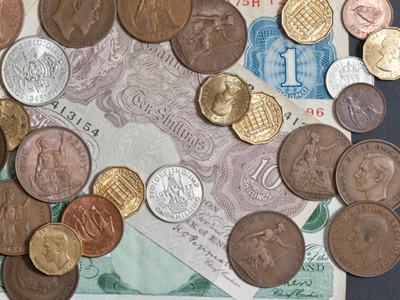Many years ago, a shilling was made up of 12 pence.

# 12 Times Table

If you are taking the 11-plus, you will need to know your times tables off by heart. This quiz is the trickiest of the times tables available in 11-Plus as it is the twelve times table. We haven't enough space for 12 questions, so have focussed on the more challenging numbers and left the easy ones (1 x 12 and 10 x 12) out. See if you can get top marks and improve your maths and numeracy skills along the way!

Learning the twelve times table is all about multiplying numbers by 12. That's not as easy as it sounds but there is a method which might help you. You need to know your two and ten times tables for this. Let's take 7 x 12 as an example. First multiply 7 x 10. This gives you the answer 70. Next multiply 7 x 2 to get 14. Finally add 70 + 14 and you should get the answer 84. Suddenly, the twelve times table doesn't look so hard after all!

Keep playing this quiz until the answers come to you without having to think. If you can master it then maths will become a whole lot easier.

1.
3 x 12 =
36
39
41
42
As well as being 12 x 3, 36 is also 6 squared
2.
5 x 12 =
48
52
54
60
To multiply a number by 5, first times it by 10 and then halve your answer
3.
12 x 9 =
106
108
110
112
12 x 10 = 120 and 120 - 12 = 108
4.
4 x 12 =
44
48
54
58
One way to multiply a number by 4 is to double it and then double your answer
5.
12 x 8 =
96
99
108
111
10 x 8 = 80 and 2 x 8 = 16. So, add 80 + 16 to get the answer
6.
12 x 11 =
121
132
144
155
This looks hard but it's really quite simple. 10 x 12 = 120 and 120 + 12 = 132
7.
6 x 12 =
72
66
54
45
There are 72 hours in 3 days
8.
12 x 7 =
71
76
78
84
Do you remember this one from the example in the introduction?
9.
12 x 2 =
14
18
24
28
2 x 12 is as simple as 12 + 12!
10.
12 x 12 =
111
121
132
144
The higher the numbers go, the harder they are to work out in your head. If you learn them over and over again, you will always know the answer. Ask any adult what 12 x 12 is and they should reply immediately. That's not because they've worked it out, but because they were taught the times tables at school too!
Author:  Sarah Garratty